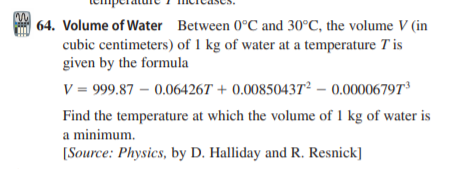# | 64. Volume of Water Between 0°C and 30°C, the volume V (in cubic centimeters) of 1 kg of water at a temperature T is given by the formula V = 999.87 – 0.06426T + 0.00850437² – 0.00006797d Find the temperature at which the volume of 1 kg of water is a minimum. [Source: Physics, by D. Halliday and R. Resnick]

Question

Volume of Water Between 0°C and 30°C, the volume V (in
cubic centimeters) of 1 kg of water at a temperature T is
given by the formulahelp_outlineImage Transcriptionclose| 64. Volume of Water Between 0°C and 30°C, the volume V (in cubic centimeters) of 1 kg of water at a temperature T is given by the formula V = 999.87 – 0.06426T + 0.00850437² – 0.00006797d Find the temperature at which the volume of 1 kg of water is a minimum. [Source: Physics, by D. Halliday and R. Resnick] fullscreen

### Want to see this answer and more?

Experts are waiting 24/7 to provide step-by-step solutions in as fast as 30 minutes!*

*Response times may vary by subject and question complexity. Median response time is 34 minutes for paid subscribers and may be longer for promotional offers.
Tagged in
Math
Algebra

### Other